### Electromagnetic Waves Class 12th Physics Part I CBSE Solution

##### Question 1.Suppose that the electric field part of an electromagnetic wave in vacuum is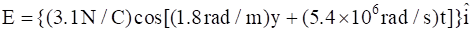(a) What is the direction of propagation?(b) What is the wavelength λ?(c) What is the frequency ν?(d) What is the amplitude of the magnetic field part of the wave?(a) Write an expression for the magnetic field part of the wave.Answer:(a) Given: E = {(3.1 N/C) cos [(1.8 rad/m) y + (5.4 × 106 rad/s)t]}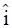As it is clear from the above electric field vector, that electric field is in the negative x direction. Therefore, the direction of propagation of the vector will be in negative y-direction i.e. –j.(b) As we know that the general equation for electric field vector in the positive x-direction is: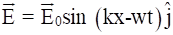..........(i)The electric field part of an electromagnetic wave in vacuum isE = {(3.1 N/C) cos [(1.8 rad /m) y + (5.4 × 106 rad/s)t..............(ii)Comparing equation (i) and (ii), we getE0 = 3.1 N(c) Angular frequency ω = 5.4 × 106 rad/sWave number i.e. k = 1.8 rad/mWavelength of the wave can be calculated as follows:λ = 2π/k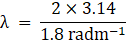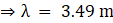(d) Angular frequency of the wave can be expressed as follows:ω = 2π v⇒ 5.4 × 106 = 2 × 3.14 × v⇒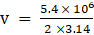⇒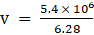⇒ V = 0.86 × 106 Hz(e) Magnetic field strength can be calculated as follows:B0 = E0/c⇒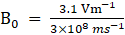⇒ B0 = 1.03 × 10-7 T(f) Since magnetic field vector is in the negative z-direction, the general equation for magnetic field vector can be written as follows:The expression for the magnetic field part of the wave can be written as follows:B = B0 cos (ky + wt) kB = {1.03 × 10-7 cos [(1.8 rad/m)y + (5.4 × 106 rad/s)t]}kQuestion 2.About 5% of the power of a 100 W light bulb is converted to visible radiation. What is the average intensity of visible radiation(a) at a distance of 1m from the bulb?(b) at a distance of 10 m?Assume that the radiation is emitted isotropically and neglect reflection.Answer:(a) Given : Power of the bulb = 100 WSince, 5% of the power of a 100 W light bulb is converted to visible radiation, therefore the power of visible radiation can be calculated as follows: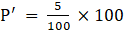P’ = 5WThe average intensity of visible radiation at a distance of 1m from the bulb can be calculated by using the following formula: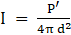⇒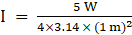⇒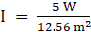⇒ I = 0.398 W/m2(b) The average intensity of visible radiation at a distance of 10 m from the bulb can be calculated by using the following formula:I = P’/4π d2I =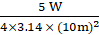I = 5/1256I = 0.00398 W/m2Question 3.Use the formula λmT = 0.29 cm K to obtain the characteristic temperature ranges for different parts of the electromagnetic spectrum. What do the numbers that you obtain tell you?Answer:According to the planck’s law of radiation:λm T = 0.29 cm Kor λm = 0.29/T cm Kwhere T is the temperature and λm is the maximum wavelength of the wavefor different values of maximum wavelengths such as λm = 10-4 cm, 5 × 10-5 cm and 10-6 cm, the values for temperatures can be calculated as follows:T = 0.29/λm cm KWhen, λm = 10-4 cmT = 0.29/10-4 cm KT = 2900 KWhen, λm = 5× 10-5 cmT =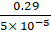cm KT = 5800 KWhen, λm = 10-6 cmT = 0.29/10-6 cm KT = 290000 KThe above data shows that temperature is required to get radiations of different parts of electromagnetic spectrum and the temperature increases with decrease in the wavelength.Question 4.Given below are some famous numbers associated with electromagnetic radiations in different contexts in physics. State the part of the electromagnetic spectrum to which each belongs.(a) 21 cm (wavelength emitted by atomic hydrogen in interstellar space).(b) 1057 MHz (frequency of radiation arising from two close energy levels in hydrogen; known as Lamb shift).(c) 2.7 K [temperature associated with the isotropic radiation filling all space-thought to be a relic of the ‘big-bang’ origin of the universe].(d) 5890 Å - 5896 Å [double lines of sodium](e) 14.4 keV [energy of a particular transition in 57Fe nucleus associated with a famous high resolution spectroscopic method (Mössbauer spectroscopy)].Answer:(a) The wavelength emitted by atomic hydrogen in interstellar space i.e. 21 cm is the radio waves as radio waves are shortest in wavelength of the electromagnetic spectrum.(b) The frequency of radiation arising from the two close energy levels in hydrogen known as Lamb shift i.e. 1057 MHz is radio waves as it belongs to the short wavelength end of the electromagnetic spectrum.(c) Given: Temperature = 2.7 Kλm = 0.29/T cm Kputting the value of temperature in above equationλm = 0.29/2.7 cm Kλm = 0.11 cmThe above wavelength belongs to the microwaves of the electromagnetic spectrum.(d) 5890 Å - 5896 Å [double lines of sodium] belongs to the yellow light of the visible spectrum of electromagnetic waves.(e) Given: Energy E = 14.4 keVEnergy is given by the relation E = hvh = 6.6 × 10-34 Jsv = E/h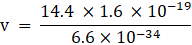⇒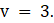4 × 1018 Hzthe electromagnetic spectrum belongs to X-rays.Question 5.Answer the following question:Long distance radio broadcasts use short-wave bands. Why?Answer:Long distance radio broadcasts use short-wave bands because ionosphere refracts only shortwave bands and long wave bands are not refracted by the ionosphere.Question 6.Answer the following question:It is necessary to use satellites for long distance TV transmission. Why?Answer:Satellites are used for long distance T.V. transmission because due to the high frequencies of the T.V. signals and high energy of the TV signals, they can’t be reflected by the ionosphere. Due to which satellites are required for reflecting the T.V. signals by the ionosphere.Question 7.Answer the following question:Optical and radio telescopes are built on the ground but X-ray astronomy is possible only from satellites orbiting the earth. Why?Answer:Optical and radio-telescopes are built on the ground because atmosphere can absorb the X-rays but visible and radio-waves are not absorbed by the atmosphere and they can penetrate through it. That’s why Optical and radio telescopes are built on the ground but X-ray astronomy is possible only from satellites orbiting the earth.Question 8.Answer the following question:The small ozone layer on top of the stratosphere is crucial for human survival. Why?Answer:The small ozone layer on top of the stratosphere is crucial for human survival because ozone layer present on top of the stratosphere helps in preventing the harmful UV radiation from reaching the Earth’s surface and absorbs it. If it reaches the Earth’s surface it can cause many serious harms to the people.Question 9.Answer the following question:If the earth did not have an atmosphere, would its average surface temperature be higher or lower than what it is now?Answer:When there will be no atmosphere on surface of Earth, then there will be no green house effect which will led to decrease in the temperature. The decrease in temperature will result in making the environment cool and it will be difficult to survive by human.Question 10.Answer the following question:Some scientists have predicted that a global nuclear war on the earth would be followed by a severe ‘nuclear winter’ with a devastating effect on life on earth. What might be the basis of this prediction?Answer:The environmental condition post nuclear war will be very worst. The war will result in formation of clouds in the sky all over resulting in the extreme winter conditions. It will prevent sunlight to reach the Earth’s surface and the Ozone layer will also gets depleted.

PDF FILE TO YOUR EMAIL IMMEDIATELY PURCHASE NOTES & PAPER SOLUTION. @ Rs. 50/- each (GST extra)

HINDI ENTIRE PAPER SOLUTION

MARATHI PAPER SOLUTION

SSC MATHS I PAPER SOLUTION

SSC MATHS II PAPER SOLUTION

SSC SCIENCE I PAPER SOLUTION

SSC SCIENCE II PAPER SOLUTION

SSC ENGLISH PAPER SOLUTION

SSC & HSC ENGLISH WRITING SKILL

HSC ACCOUNTS NOTES

HSC OCM NOTES

HSC ECONOMICS NOTES

HSC SECRETARIAL PRACTICE NOTES

# 2019 Board Paper Solution

HSC ENGLISH SET A 2019 21st February, 2019

HSC ENGLISH SET B 2019 21st February, 2019

HSC ENGLISH SET C 2019 21st February, 2019

HSC ENGLISH SET D 2019 21st February, 2019

SECRETARIAL PRACTICE (S.P) 2019 25th February, 2019

HSC XII PHYSICS 2019 25th February, 2019

CHEMISTRY XII HSC SOLUTION 27th, February, 2019

OCM PAPER SOLUTION 2019 27th, February, 2019

HSC MATHS PAPER SOLUTION COMMERCE, 2nd March, 2019

HSC MATHS PAPER SOLUTION SCIENCE 2nd, March, 2019

SSC ENGLISH STD 10 5TH MARCH, 2019.

HSC XII ACCOUNTS 2019 6th March, 2019

HSC XII BIOLOGY 2019 6TH March, 2019

HSC XII ECONOMICS 9Th March 2019

SSC Maths I March 2019 Solution 10th Standard11th, March, 2019

SSC MATHS II MARCH 2019 SOLUTION 10TH STD.13th March, 2019

SSC SCIENCE I MARCH 2019 SOLUTION 10TH STD. 15th March, 2019.

SSC SCIENCE II MARCH 2019 SOLUTION 10TH STD. 18th March, 2019.

SSC SOCIAL SCIENCE I MARCH 2019 SOLUTION20th March, 2019

SSC SOCIAL SCIENCE II MARCH 2019 SOLUTION, 22nd March, 2019

XII CBSE - BOARD - MARCH - 2019 ENGLISH - QP + SOLUTIONS, 2nd March, 2019

# HSCMaharashtraBoardPapers2020

(Std 12th English Medium)

HSC ECONOMICS MARCH 2020

HSC OCM MARCH 2020

HSC ACCOUNTS MARCH 2020

HSC S.P. MARCH 2020

HSC ENGLISH MARCH 2020

HSC HINDI MARCH 2020

HSC MARATHI MARCH 2020

HSC MATHS MARCH 2020

# SSCMaharashtraBoardPapers2020

(Std 10th English Medium)

English MARCH 2020

HindI MARCH 2020

Hindi (Composite) MARCH 2020

Marathi MARCH 2020

Mathematics (Paper 1) MARCH 2020

Mathematics (Paper 2) MARCH 2020

Sanskrit MARCH 2020

Sanskrit (Composite) MARCH 2020

Science (Paper 1) MARCH 2020

Science (Paper 2)

Geography Model Set 1 2020-2021

MUST REMEMBER THINGS on the day of Exam

Are you prepared? for English Grammar in Board Exam.

Paper Presentation In Board Exam

How to Score Good Marks in SSC Board Exams

Tips To Score More Than 90% Marks In 12th Board Exam

How to write English exams?

How to prepare for board exam when less time is left

How to memorise what you learn for board exam

No. 1 Simple Hack, you can try out, in preparing for Board Exam

How to Study for CBSE Class 10 Board Exams Subject Wise Tips?

JEE Main 2020 Registration Process – Exam Pattern & Important Dates

NEET UG 2020 Registration Process Exam Pattern & Important Dates

How can One Prepare for two Competitive Exams at the same time?

8 Proven Tips to Handle Anxiety before Exams!